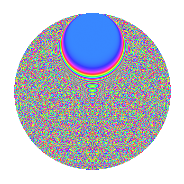# Properties

 Label 2541.2.byLevel 2541 Weight 2 Character orbit by Rep. character $$\chi_{2541}(13,\cdot)$$ Character field $$\Q(\zeta_{110})$$ Dimension 7040 Sturm bound 704

# Related objects

## Defining parameters

 Level: $$N$$ = $$2541 = 3 \cdot 7 \cdot 11^{2}$$ Weight: $$k$$ = $$2$$ Character orbit: $$[\chi]$$ = 2541.by (of order $$110$$ and degree $$40$$) Character conductor: $$\operatorname{cond}(\chi)$$ = $$847$$ Character field: $$\Q(\zeta_{110})$$ Sturm bound: $$704$$

## Dimensions

The following table gives the dimensions of various subspaces of $$M_{2}(2541, [\chi])$$.

Total New Old
Modular forms 14240 7040 7200
Cusp forms 13920 7040 6880
Eisenstein series 320 0 320

## Trace form

 $$7040q - 172q^{4} - 10q^{7} + 20q^{8} + 1760q^{9} + O(q^{10})$$ $$7040q - 172q^{4} - 10q^{7} + 20q^{8} + 1760q^{9} - 28q^{11} - 48q^{14} + 12q^{15} + 192q^{16} - 10q^{18} + 84q^{22} + 68q^{23} - 204q^{25} + 30q^{28} + 40q^{29} + 40q^{35} + 172q^{36} + 92q^{37} + 6q^{42} - 44q^{44} + 70q^{46} - 2q^{49} + 40q^{51} - 192q^{53} - 48q^{56} + 24q^{58} + 24q^{60} - 10q^{63} - 232q^{64} + 64q^{67} + 42q^{70} - 36q^{71} + 10q^{72} - 80q^{74} - 90q^{77} + 24q^{78} - 128q^{79} - 1760q^{81} - 60q^{84} + 128q^{85} + 46q^{86} - 96q^{88} + 12q^{91} + 422q^{92} + 36q^{93} - 20q^{95} + 48q^{99} + O(q^{100})$$

## Decomposition of $$S_{2}^{\mathrm{new}}(2541, [\chi])$$ into newform subspaces

The newforms in this space have not yet been added to the LMFDB.

## Decomposition of $$S_{2}^{\mathrm{old}}(2541, [\chi])$$ into lower level spaces

$$S_{2}^{\mathrm{old}}(2541, [\chi]) \cong$$ $$S_{2}^{\mathrm{new}}(847, [\chi])$$$$^{\oplus 2}$$

## Hecke Characteristic Polynomials

There are no characteristic polynomials of Hecke operators in the database